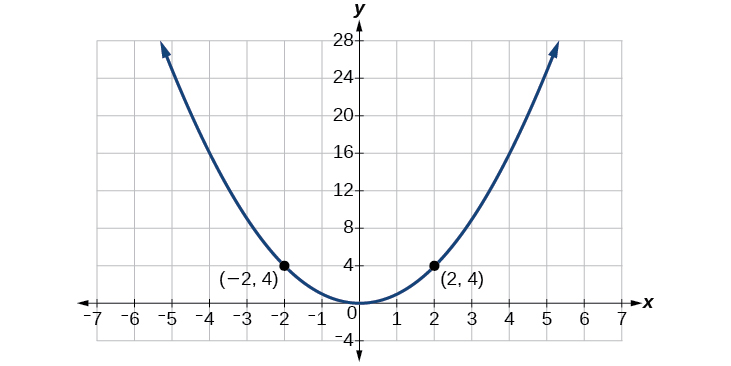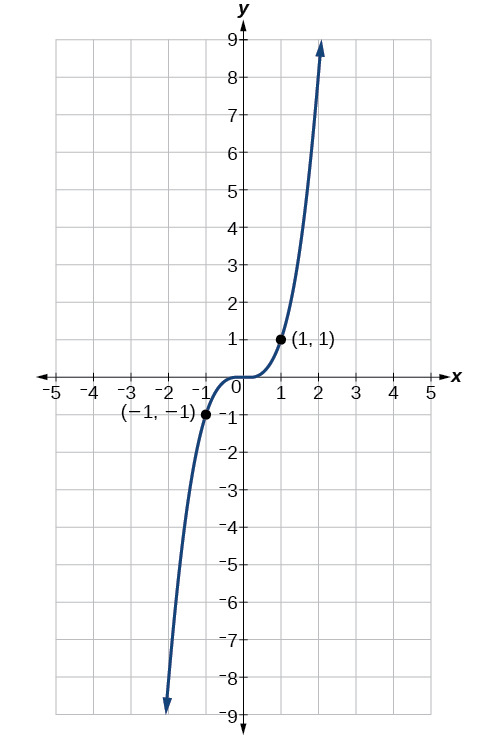# 7.4 The other trigonometric functions  (Page 3/14)

 Page 3 / 14

Use reference angles to find all six trigonometric functions of $\text{\hspace{0.17em}}-\frac{7\pi }{4}.$

$\begin{array}{l}\\ \mathrm{sin}\left(\frac{-7\pi }{4}\right)=\frac{\sqrt{2}}{2},\mathrm{cos}\left(\frac{-7\pi }{4}\right)=\frac{\sqrt{2}}{2},\mathrm{tan}\left(\frac{-7\pi }{4}\right)=1,\\ \mathrm{sec}\left(\frac{-7\pi }{4}\right)=\sqrt{2},\mathrm{csc}\left(\frac{-7\pi }{4}\right)=\sqrt{2},\mathrm{cot}\left(\frac{-7\pi }{4}\right)=1\end{array}$

## Using even and odd trigonometric functions

To be able to use our six trigonometric functions freely with both positive and negative angle inputs, we should examine how each function treats a negative input. As it turns out, there is an important difference among the functions in this regard.

Consider the function $\text{\hspace{0.17em}}f\left(x\right)={x}^{2},$ shown in [link] . The graph of the function is symmetrical about the y -axis. All along the curve, any two points with opposite x -values have the same function value. This matches the result of calculation: $\text{\hspace{0.17em}}{\left(4\right)}^{2}={\left(-4\right)}^{2},{\left(-5\right)}^{2}={\left(5\right)}^{2},$ and so on. So $\text{\hspace{0.17em}}f\left(x\right)={x}^{2}\text{\hspace{0.17em}}$ is an even function, a function such that two inputs that are opposites have the same output. That means $\text{\hspace{0.17em}}f\left(-x\right)=f\left(x\right).$The function   f ( x ) = x 2   is an even function.

Now consider the function $\text{\hspace{0.17em}}f\left(x\right)={x}^{3},$ shown in [link] . The graph is not symmetrical about the y -axis. All along the graph, any two points with opposite x -values also have opposite y -values. So $\text{\hspace{0.17em}}f\left(x\right)={x}^{3}\text{\hspace{0.17em}}$ is an odd function, one such that two inputs that are opposites have outputs that are also opposites. That means $\text{\hspace{0.17em}}f\left(-x\right)=-f\left(x\right).$The function   f ( x ) = x 3   is an odd function.

We can test whether a trigonometric function is even or odd by drawing a unit circle with a positive and a negative angle, as in [link] . The sine of the positive angle is $\text{\hspace{0.17em}}y.\text{\hspace{0.17em}}$ The sine of the negative angle is $\text{\hspace{0.17em}}-y.\text{\hspace{0.17em}}$ The sine function, then, is an odd function. We can test each of the six trigonometric functions in this fashion. The results are shown in [link] .

## Even and odd trigonometric functions

An even function is one in which $\text{\hspace{0.17em}}f\left(-x\right)=f\left(x\right).$

An odd function is one in which $\text{\hspace{0.17em}}f\left(-x\right)=-f\left(x\right).$

Cosine and secant are even:

Sine, tangent, cosecant, and cotangent are odd:

## Using even and odd properties of trigonometric functions

If the secant of angle $\text{\hspace{0.17em}}t\text{\hspace{0.17em}}$ is 2, what is the secant of $\text{\hspace{0.17em}}-t?$

Secant is an even function. The secant of an angle is the same as the secant of its opposite. So if the secant of angle $\text{\hspace{0.17em}}t\text{\hspace{0.17em}}$ is 2, the secant of $\text{\hspace{0.17em}}-t\text{\hspace{0.17em}}$ is also 2.

If the cotangent of angle $\text{\hspace{0.17em}}t\text{\hspace{0.17em}}$ is $\text{\hspace{0.17em}}\sqrt{3},$ what is the cotangent of $\text{\hspace{0.17em}}-t?$

$-\sqrt{3}$

## Recognizing and using fundamental identities

We have explored a number of properties of trigonometric functions. Now, we can take the relationships a step further, and derive some fundamental identities. Identities are statements that are true for all values of the input on which they are defined. Usually, identities can be derived from definitions and relationships we already know. For example, the Pythagorean Identity    we learned earlier was derived from the Pythagorean Theorem and the definitions of sine and cosine.

## Fundamental identities

We can derive some useful identities    from the six trigonometric functions. The other four trigonometric functions can be related back to the sine and cosine functions using these basic relationships:

$\mathrm{tan}\text{\hspace{0.17em}}t=\frac{\mathrm{sin}\text{\hspace{0.17em}}t}{\mathrm{cos}\text{\hspace{0.17em}}t}$
$\mathrm{sec}\text{\hspace{0.17em}}t=\frac{1}{\mathrm{cos}\text{\hspace{0.17em}}t}$
$\mathrm{csc}\text{\hspace{0.17em}}t=\frac{1}{\mathrm{sin}\text{\hspace{0.17em}}t}$
$\text{cot}\text{\hspace{0.17em}}t=\frac{1}{\text{tan}\text{\hspace{0.17em}}t}=\frac{\text{cos}\text{\hspace{0.17em}}t}{\text{sin}\text{\hspace{0.17em}}t}$

bsc F. y algebra and trigonometry pepper 2
given that x= 3/5 find sin 3x
4
DB
remove any signs and collect terms of -2(8a-3b-c)
-16a+6b+2c
Will
Joeval
(x2-2x+8)-4(x2-3x+5)
sorry
Miranda
x²-2x+9-4x²+12x-20 -3x²+10x+11
Miranda
x²-2x+9-4x²+12x-20 -3x²+10x+11
Miranda
(X2-2X+8)-4(X2-3X+5)=0 ?
master
The anwser is imaginary number if you want to know The anwser of the expression you must arrange The expression and use quadratic formula To find the answer
master
The anwser is imaginary number if you want to know The anwser of the expression you must arrange The expression and use quadratic formula To find the answer
master
Y
master
master
Soo sorry (5±Root11* i)/3
master
Mukhtar
explain and give four example of hyperbolic function
What is the correct rational algebraic expression of the given "a fraction whose denominator is 10 more than the numerator y?
y/y+10
Mr
Find nth derivative of eax sin (bx + c).
Find area common to the parabola y2 = 4ax and x2 = 4ay.
Anurag
A rectangular garden is 25ft wide. if its area is 1125ft, what is the length of the garden
to find the length I divide the area by the wide wich means 1125ft/25ft=45
Miranda
thanks
Jhovie
What do you call a relation where each element in the domain is related to only one value in the range by some rules?
A banana.
Yaona
given 4cot thither +3=0and 0°<thither <180° use a sketch to determine the value of the following a)cos thither
what are you up to?
nothing up todat yet
Miranda
hi
jai
hello
jai
Miranda Drice
jai
aap konsi country se ho
jai
which language is that
Miranda
I am living in india
jai
good
Miranda
what is the formula for calculating algebraic
I think the formula for calculating algebraic is the statement of the equality of two expression stimulate by a set of addition, multiplication, soustraction, division, raising to a power and extraction of Root. U believe by having those in the equation you will be in measure to calculate it
Miranda
state and prove Cayley hamilton therom
hello
Propessor
hi
Miranda
the Cayley hamilton Theorem state if A is a square matrix and if f(x) is its characterics polynomial then f(x)=0 in another ways evey square matrix is a root of its chatacteristics polynomial.
Miranda
hi
jai
hi Miranda
jai
thanks
Propessor
welcome
jai
What is algebra
algebra is a branch of the mathematics to calculate expressions follow.
Miranda
Miranda Drice would you mind teaching me mathematics? I think you are really good at math. I'm not good at it. In fact I hate it. 😅😅😅
Jeffrey
lolll who told you I'm good at it
Miranda
something seems to wispher me to my ear that u are good at it. lol
Jeffrey
lolllll if you say so
Miranda
but seriously, Im really bad at math. And I hate it. But you see, I downloaded this app two months ago hoping to master it.
Jeffrey
which grade are you in though
Miranda
oh woww I understand
Miranda
Jeffrey
Jeffrey
Miranda
how come you finished in college and you don't like math though
Miranda
gotta practice, holmie
Steve
if you never use it you won't be able to appreciate it
Steve
I don't know why. But Im trying to like it.
Jeffrey
yes steve. you're right
Jeffrey
so you better
Miranda
what is the solution of the given equation?
which equation
Miranda
I dont know. lol
Jeffrey
Miranda
JeffreyByByBy Janet ForresterByBy Madison ChristianBy OpenStaxBy Richley CrapoBy Ryan LoweBy OpenStaxBy Prateek AshtikarBy Yasser IbrahimBy Jemekia Weeden